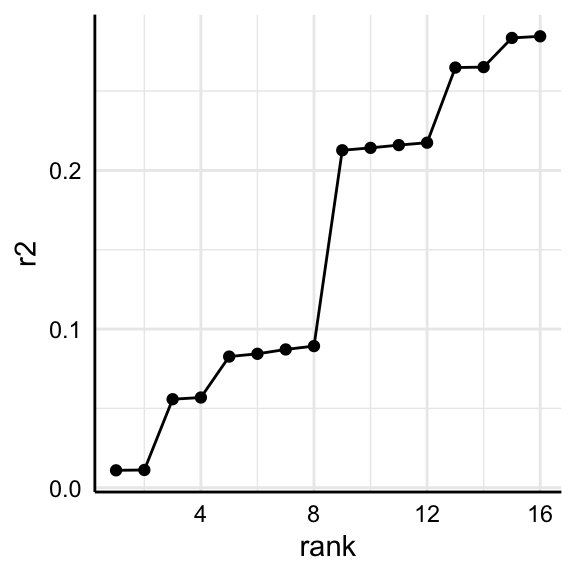# Investigating specific specifications

Per default, specr() summarizes individual specifications by using broom::tidy() and broom::glance(). For most cases, this provides a sufficient and appropriate summary of the relationship of interest and model characteristics. Sometimes, however, it might be useful to investigate specific models in more detail or to investigate a specific parameter that is not provided by the two functions (e.g., r-square). This vignette shows how to access individual models and extract further information from them.

library(tidyverse)
library(specr)
library(performance)

## Setup specifications with a specific extract function

If we want to investigate individual models and particularly all aspects of that model, we need to create a custom extract function that also stores the entire model object in the result data frame.

# Custom function
tidy_new <- function(x) {
fit <- broom::tidy(x, conf.int = TRUE)
fit$res <- list(x) # Store model object return(fit) } # Run specification curve analysis specs <- setup(data = example_data, y = c("y1", "y2"), x = c("x1", "x2"), model = c("lm"), controls = c("c1", "c2"), subsets = list(group1 = unique(example_data$group1),
group2 = unique(example_data\$group2)),
fun1 = tidy_new)

results <- specr(specs)

## Identify model(s) of interest

For this example, we are going to look at two specific models (same independent variables, all controls, all participants, but different dependent variables).

(y_models <- results %>%
as_tibble %>%
filter(x == "x1",
controls == "c1 + c2",
subsets == "all")) %>%
select(x:group2, estimate:res)
#> # A tibble: 2 × 14
#>   x     y     model controls subsets group1 group2 estimate std.error statistic
#>   <chr> <chr> <chr> <chr>    <chr>   <fct>  <fct>     <dbl>     <dbl>     <dbl>
#> 1 x1    y1    lm    c1 + c2  all     <NA>   <NA>      0.593    0.0393     15.1
#> 2 x1    y2    lm    c1 + c2  all     <NA>   <NA>     -0.214    0.0389     -5.51
#> # … with 4 more variables: p.value <dbl>, conf.low <dbl>, conf.high <dbl>,
#> #   res <list>

As you can see, the resulting tibble includes an additional column called res. This column includes the entire “model object” and we can use it to further investigate each model.

## Investigate models

For example, we can now easily get a full summary of the models and compare individual coefficients and statistics.

y_models %>%
pull(res) %>%
map(summary) %>%
map(coef)
#> []
#>                Estimate Std. Error    t value      Pr(>|t|)
#> (Intercept) -1.09466509 0.03582021 -30.559989 3.127670e-145
#> x1           0.59291826 0.03933279  15.074403  2.084917e-46
#> c1           0.06781034 0.03327698   2.037756  4.183823e-02
#> c2          -0.04278267 0.03079000  -1.389499  1.649916e-01
#>
#> []
#>                Estimate Std. Error   t value     Pr(>|t|)
#> (Intercept)  0.79394670 0.03540987 22.421621 1.828378e-90
#> x1          -0.21413105 0.03888221 -5.507172 4.640637e-08
#> c1           0.03921893 0.03289578  1.192218 2.334599e-01
#> c2           0.54186302 0.03043728 17.802607 9.085852e-62

Or we could get r-squared values for both models (here using the function r2() from the performance package).

y_models %>%
pull(res) %>%
map(r2)       # r2 is include in the package "performance"
#> []
#> # R2 for Linear Regression
#>        R2: 0.217
#>
#> []
#> # R2 for Linear Regression
#>        R2: 0.285
#>   adj. R2: 0.282

## Some more examples

This way, we can analyze or compare such statistics across several models.

r2_results <- results %>%
as_tibble %>%
filter(subsets == "all") %>%
mutate(r2 = map(res, r2),
r2 = map_dbl(r2, 1)) %>%
arrange(r2)

r2_results %>%
select(x:controls, r2)
#> # A tibble: 16 × 5
#>    x     y     model controls          r2
#>    <chr> <chr> <chr> <chr>          <dbl>
#>  1 x2    y2    lm    no covariates 0.0110
#>  2 x2    y2    lm    c1            0.0112
#>  3 x1    y2    lm    no covariates 0.0558
#>  4 x1    y2    lm    c1            0.0569
#>  5 x2    y1    lm    no covariates 0.0827
#>  6 x2    y1    lm    c2            0.0844
#>  7 x2    y1    lm    c1            0.0872
#>  8 x2    y1    lm    c1 + c2       0.0893
#>  9 x1    y1    lm    no covariates 0.213
#> 10 x1    y1    lm    c2            0.214
#> 11 x1    y1    lm    c1            0.216
#> 12 x1    y1    lm    c1 + c2       0.217
#> 13 x2    y2    lm    c2            0.265
#> 14 x2    y2    lm    c1 + c2       0.265
#> 15 x1    y2    lm    c2            0.284
#> 16 x1    y2    lm    c1 + c2       0.285

And we can plot comparisons…

r2_results %>%
arrange(r2) %>%
mutate(rank = 1:n()) %>%
ggplot(aes(x = rank,
y = r2)) +
geom_point() +
geom_line() +
theme_minimal() +
theme(strip.text = element_blank(),
axis.line = element_line("black", size = .5),
axis.text = element_text(colour = "black"))